# Volume and Surface area – Concepts and Formula

## Volume and Surface area – Concepts and Formula

Surface area and volume are calculated for any three-dimensional geometrical shape. The surface area of any given object is the area or region occupied by the surface of the object. Whereas volume is the amount of space available in an object.

#### Total surface area

Total surface area refers to the area including the base(s) and the curved part. It is total of the area covered by the surface of the object. If the shape has curved surface and base, then total area will be the sum of the two areas.

#### Curved surface area/Lateral surface area

Curved surface area refers to the area of only the curved part of the shape excluding its base(s). It is also referred to as lateral surface area for shapes such as a cylinder.

#### Volume

The amount of space, measured in cubic units, that an object or substance occupies is called volume. Two-dimensional doesn’t have volume but has area only. For example, Volume of Circle cannot be found, though Volume of the sphere can be. It is so because a sphere is a three-dimensional shape.

####Let each edge of a cube be of length a, then

Volume = (side)3 = a3 cubic units.
Surface Area = 6 x (Area of one face )2 = 6a2 sq. units.#### CuboidLet length = l, breadth = b and height = h units. Then,
Volume = length x breadth x height = ( l x b x h ) cubic units

Surface area = 2 (lb + bh + lh) sq. units.####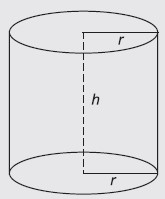Let radius of base = r and Height (or length) = h. Then,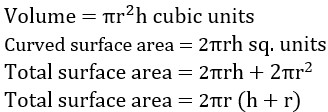####Let radius of base = r and Height = h. Then,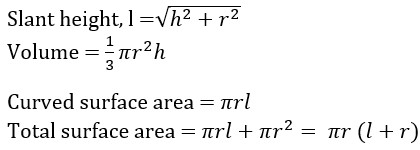#### Sphere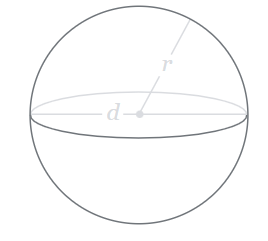Let the radius of the sphere be r. Then,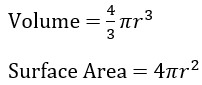#### Hemi sphere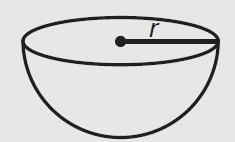Question Find the volume and surface area of a cuboid 12 m long, 8 m broad and 5 m high.
Answer.Volume = 12 x 8 x 5 = 480 m3
Surface area = 2 ( 12 x 8 + 8 x 5 + 5 x 12 )
= 2 ( 96 + 40 + 60 )
= 2 (196)
= 392

QuestionsThe diagonal of a cube is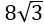cm. Find its volume and surface area.
Let the edge of the cube be a.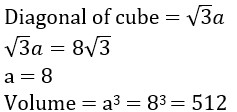Surface Area = 6a2 = 6.82 = 6 x 64 = 384

Question. A rectangular water reservoir contains 42000 litres of water. If the length of reservoir is 6 m and breadth of the reservoir is 3.5 m, then the depth of the reservoir will be ?
Let the depth be h
Volume = 6 x 3.5 x h
42 = 21 x h
h = 42 / 21 = 2 m

Question. The radii of two spheres are in the ratio 3 : 2. What is ratio of their volume ?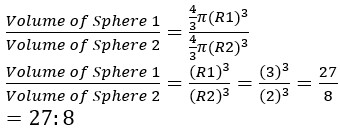#### Attempt Quantitative Aptitude Topic Wise Online Test Series

Recommended PDF’s for :

#### Most important PDF’s for Bank, SSC, Railway and Other Government Exam : Download PDF Now

AATMA-NIRBHAR Series- Static GK/Awareness Practice Ebook PDF Get PDF here
The Banking Awareness 500 MCQs E-book| Bilingual (Hindi + English) Get PDF here
AATMA-NIRBHAR Series- Banking Awareness Practice Ebook PDF Get PDF here
Computer Awareness Capsule 2.O Get PDF here
AATMA-NIRBHAR Series Quantitative Aptitude Topic-Wise PDF Get PDF here
AATMA-NIRBHAR Series Reasoning Topic-Wise PDF Get PDF Here
Memory Based Puzzle E-book | 2016-19 Exams Covered Get PDF here
Caselet Data Interpretation 200 Questions Get PDF here
Puzzle & Seating Arrangement E-Book for BANK PO MAINS (Vol-1) Get PDF here
ARITHMETIC DATA INTERPRETATION 2.O E-book Get PDF here
3